• 最小二乘法参数辨识
2021-04-18 04:25:15

function [sysd,sys,err] = ID(Y,U,Ts)

%

%基于递推最小二乘法的参数辨识程序

%仅针对二阶系统：)

%出处：http://blog.sina.com.cn/xianfa110

%=-=-=-=-=-=-=-=-=-=-==-=-=-=-=-=

%Inputs:

%=-=-=-=-=-=-=-=-=-=-==-=-=-=-=-=

%Y = nX1 vector of your model output

%U = nX1 vector of your model input

%Ts = sample time

%

%=-=-=-=-=-=-=-=-=-=-==-=-=-=-=-=

%Outputs:

%=-=-=-=-=-=-=-=-=-=-==-=-=-=-=-=

%sysd = discrete-time transfer function identified

%sys = continuous-time transfer function identified

%err = error

%

if nargin<3 || nargin>3

error('Must be three inputs!');

end

if length(Y)~=length(U)

error('length of inputs must be equal.');

end

n=length(U);

Y=reshape(Y,n,1);

U=reshape(U,n,1);

theta=[0.1;0.1;0.1;0.1;0.1];

P=2^25*eye(5);

R0=1;

for m=3:n

X=[Y(m-1) Y(m-2) U(m) U(m-1) U(m-2)]';

alfa=1/(R0+X'*P*X);

L=alfa*P*X;

theta(:,m-1)=theta(:,m-2)+L*(Y(m)-X'*theta(:,m-2));

P=P/R0-alfa*P*X*X'*P;

err=Y(m)-X'*theta(:,m-2);

if abs(err)<=1e-10

break;

end

end

m=length(theta(1,:));

result=[-theta(1:2,m);theta(3:5,m)];

t=1:m;

figure;

plot(t,theta(1,:),t,theta(2,:),t,theta(3,:),t,theta(4,:),t,theta(5,:));

legend('th1','th2','th3','th4','th5');

num=[result(3),result(4),result(5)];

den=[1,result(1),result(2)];

sysd=tf(num,den,Ts);

[n,d]=d2cm(num,den,Ts,'tustin');

sys=tf(n,d);

%%====================================================

exaple:

对于以下模型：输入以下代码：

Y=data(:,3);U=data(:,2);

[sysd,sys,e]=ID(Y,U,0.001);

得到结果如下：

Transfer function:

-4.314e-009 z^2 + 8.784e-005 z + 4.362e-005

-------------------------------------------

z^2 - 1.975

z + 0.9753

Sampling time: 0.001

Transfer function:

-1.12e-005 s^2 - 0.04417 s + 133.1

----------------------------------

s^2 + 25.02 s - 0.008906

e =

-6.8120e-011

相关文章：

更多相关内容
• 基于MATLAB的最小二乘法参数辨识与仿真.pdf
• RLS参数辨识最小二乘法RLS参数辨识最小二乘法RLS参数辨识最小二乘法
• 资源名：最小二乘法参数辨识方法_电动汽车动力电池参数辨识模型_动力电池参数辨识模型_SOC_matlab仿真 资源类型：matlab项目全套源码 源码说明： 全部项目源码都是经过测试校正后百分百成功运行的，如果您下载后不能...
• 针对电池一阶模型，使用最小二乘法进行参数辨识，使误差最小。有研究电池模型或者最小二乘法的同学可以过来看看。
• 系统参数辨识常用的是最小二乘法，但最小二乘法存在限制需要改进，该包中含常用的方法如递推最小二乘法，遗忘因子最小二乘法，增广最小二乘法，辅助变量法的matlab代码
• 基于改进的PI模型对非线性曲线进行拟合，二次寻优算法进行参数辨识，用逆模型前馈补偿
• 基于MATLAB的递推最小二乘法辨识与仿真.pdf

关键词：永磁同步电机;参数辨识;最小二乘法

Simulation of PMSM based on least squares on-line parameter identification

WANG Hong-shan , ZHANG Xing，XIE Zhen , YANG Shu-ying

Abstract：This paper presents a bbbbbb to determine the parameters of PMSM on line which are necessary to implement the vector control strategy. The presented identification technique, based least-squares, reveals itself suitable to be applied to PMSM. The estimation is based on a standard model of PMSM, expressed in rotor coordinates. The bbbbbb is suitable for online operation to continuously update the parameter values. The developed algorithm is simulated in MATLAB/SIMULINK. Simulation results are presented, and accurate parameters for PMSM is provided.

KEY WORDS：PMSM; Parameter Identification; Least-Squares

0 引言

电机参数辨识方面的文献数量颇多，研究成果丰富。参数辨识技术的研究始于70年代末、80年代初。直至今日，这个领域仍有新的研究成果出现、参数辨识的方法众多，方法与方法之间各有不同。但总的说来，现有的辨识方法可以归纳成5种类型，分别是：信号注入法、直接估算法、补偿坐标系法、最小二乘法、模型参考自适应方法。

1 基于最小二乘法的永磁同步电机参数辨识

1.1 电机参数辨识的必要性

在交流调速系统中，矢量控制技术能使交流电动机获得与他励直流电动机一样的控制特性，采用矢量控制技术的交流调速系统的性能达到直流调速系统的水平，无速度传感器的矢量控制是在常规带速度传感器的矢量控制基础上发展起来的，在无速度传感器的矢量控制中必须用到电机参数。工程上不能预知现场所用电机的参数，也不可能采用常规的空载试验和堵转试验去测量电机参数，并且随着电机的老化和周围环境的变化，电机实际参数与所给参数之间存在较大的差别，所以作为通用变频器必须具有电机参数的自测定功能。电机投入正常运行之前的参数辨识方法，特别是不需要增加任何附加电路而仅靠电机调速系统本身实现参数辨识方法已成为现代交流电机参数辨识的新特点。从控制的角度来讲, 解决受控对象参数不准的方法之一就是对受控对象的参数进行在线辨识,并不断更新其参数值, 使控制器设定值与实际值相适应。

1.2 最小二乘法的基本原理

最小二乘法最初是由解超定方程，求最优解提出的。设y为一组自变量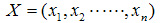的函数，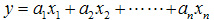。若进行m次观测，则：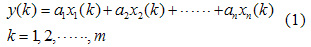但m=n时，只要A-1存在，即可求出待定参数：a=(a1,a2...,an)，上式有唯一解：a=A-1y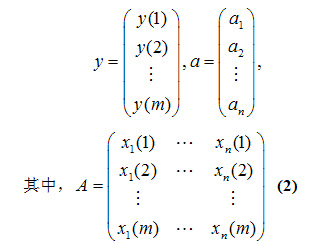当m>n时，称为超定方程组，通常不能选定一组参数以满足所有的m个方程，因而需要用估计方法估算最佳值。可以采用最小二乘法进行估计：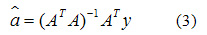1.3 基于最小二乘法的永磁同步电机参数辨识

在基于最小二乘法的电机参数辨识中关键在于如何得到以待辨识参数为未知量的线性方程。下面说明如何在转子同步旋转坐标系下获得以待辨识参数为未知量线性方程的电机模型。

首先用固定于转子的同步旋转坐标dq轴系来分析永磁同步电机的数学模型。取转子永磁体基波励磁磁场轴线(磁极轴线)为d轴，d轴与A相绕组的夹角θr，而q轴(交轴)逆时针方向超前d轴90度电角度，xy坐标系为固定在定子上的旋转坐标系，定子磁链的方向为x轴的正方向，dq轴随同转子以电角速度ωr一起旋转。dq轴上的分量可以由定子三相绕组经三相坐标系或矢量变换得到，即进行三相轴系到两相旋转轴系dq的变换。

以电流的旋转变换为例分析如何得到转子同步旋转坐标系下的电机模型。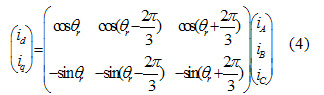式中， θr为转子位置;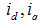为定子的d轴和q轴电流;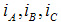为A轴、B轴和C轴的电流。

通过式(4)的坐标变换可以得到在转子同步旋转坐标系下电机的模型方程：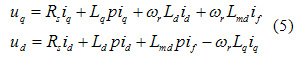式中： Rs为定子电阻; Ld为d轴电感; Lq为q轴电感; Lmd为励磁电感; if为等效励磁电流。

在电机中，若不计温度变化对永磁体的供磁的影响，可认为永磁体的基波磁场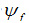是恒定的，即if是个常量。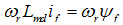，实际上是d轴永磁体励磁磁场在q轴线圈中产生的运动电动势，也就是空载电动势e0。式(5)可以化简为：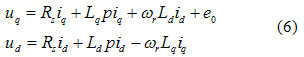式(6)写成矩阵形式：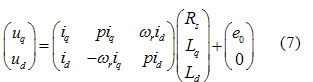由式(7)可以明显地看出，经过了坐标变换得到了以定子电阻Rs、q轴电感La和d轴电感Ld为未知量的线性方程，可以运用最小二乘法对电机参数进行辨识。

2 基于最小二乘法永磁同步电机参数辨识的仿真模型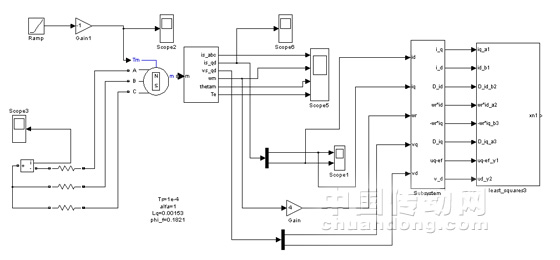图1 基于最小二乘法参数辨识的系统原理框图

系统采用控制方案：根据模块化建模的思想，将控制系统分割为各个功能独立的子模块，其中主要包括：永磁同步电机本体模块、电机运行状态测量模块和最小二乘法参数辨识模块。

3 仿真结果

针对上述建立的仿真模型进行了永磁同步电机系统的仿真测试。永磁同步电机参数见表1：

表1 仿真参数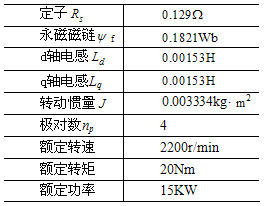仿真的过程中，在永磁同步电机的转子轴上加-30Nm/s递增的转矩，使永磁同步运行在发电模式的同时，电机处于不停地加速状态，这样做的目的是使得电机的状态方程满足式(7)。仿真的最大步长为 ，采用M语言编写的参数辨识模块于0.53s启动，每隔0.01s进行一次参数辨识。从而每隔0.01s利用新辨识出的电机参数在之前辨识出的电机参数的基础上进行更新，以获得准确的实时电机参数。

图2是在电机待辨识参数初始值都为0的情况下，对永磁同步电机控制过程中参数辨识的仿真结果：虚线分别表示电机的实际参数值，实线表示参数辨识的结果。从图2的a、b和c图都可以看出从0.53s算法开始运行，永磁电机的d轴和q轴电感包括定子电阻从初始值0开始单调地向电机相应的实际参数进行收敛，并无限地逼近相应电机参数的实际值。

仿真结果表明基于最小二乘法的参数辨识算法能够在线实时准确辨识出电机参数，具有较好的收敛性和辨识精度。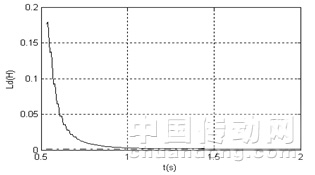(a)d轴电感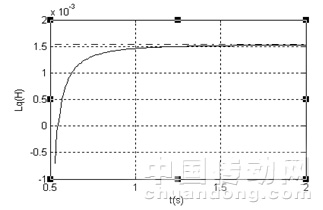(b)q轴电感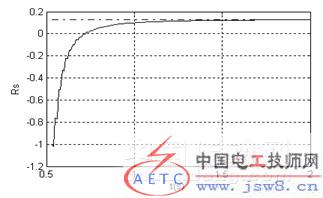(c) 定子电阻

图2 电机参数辨识结果

4 结 论

展开全文• 最小二乘参数辨识，是同济大学汽车学院的系统辨识课件，126页，看着不错，希望大家分享
• 仅适合初学者 包含四个程序 递推最小二乘法、递推增广最小二乘法、遗忘因子递推最小二乘法、遗忘因子递推增广最小二乘法
• nbspmatlab基于MATLAB最小二乘法系统辨识与仿真.pdf6页本文档一共被下载：次,您可全文免费在线阅读后下载本文档。 下载提示1.本站不保证该用户上传的文档完整性，不预览、不比对内容而直接下载产生的反悔问题本站...

您所在位置：网站首页 > 海量文档

&nbsp>&nbsp计算机&nbsp>&nbspmatlab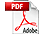基于MATLAB最小二乘法系统辨识与仿真.pdf6页

本文档一共被下载：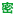次,您可全文免费在线阅读后下载本文档。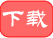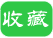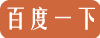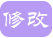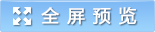下载提示

1.本站不保证该用户上传的文档完整性，不预览、不比对内容而直接下载产生的反悔问题本站不予受理。

2.该文档所得收入(下载+内容+预览三)归上传者、原创者。

3.登录后可充值，立即自动返金币，充值渠道很便利

第29卷第2期 许昌学院学报 V01．29．No．2

OFXUCHANGUNIVERSITY Mar．2010

2010年3月 JOURNAL

文章编号：167l一9824120lO)02一0024一04

基于MATLAB的最小二乘法系统辨识与仿真

郭利辉1，朱励洪2，高 巍2

摘 要：将MATLAB应用到系统辨识中，在分析最小二乘法的基本原理和推导过程的基础

上给出了系统辩识中算法参数估计的递推公式，并进行了实例仿真．

关键词：系统辨识；最小二乘；MATLAB

中图分类号：TP273 文献标识码：A

辨识、状态估计和控制理论是现代控制理论三个互相渗透的领域．利用控制理论去解决实际问题时，

给出“辨识”的定义为：系统辨识是在对输入和输出观测的基础上，在指定的一类系统中，确定一个与被识

示客观系统(或将要构造的系统)本质特征的一种演算，并用这个模型把对客观系统的理解表示成有用的

形式”[2】．1978年，L．Ljung给出“辨识”更加实用的定义：“辨识有三个要素——数据，模型类和准则．辨识

就是按照一个准则在一组模型类中选择一个与数据拟合得最好的模型”旧1．总而言之，辨识的实质就是从

一组模型类中选择一个模型，按照某种准则，使之能最好地拟合所关心的实际过程的静态或动态特性．经

典的系统辨识方法有p“】：阶跃响应法、脉冲响应法、频率响应法、相关分析法、谱分析法、最小二乘法和极

大似然法等，其中最小二乘法(LS)是一种经典的和最基本的，也是应用最广泛的方法，可用于动态静态、

线性、非线性系统．

MATLAB具有很强大的运算能力，特别是在向量、数组、矩阵运算等方面，以及求解高次方程、常微分

方程数值积分运算、最优化方法等方面具有独特的特点，另外，MATLAB还具有精密的图形绘制的特点，

使得运算结果能够更加直观的呈现出来一】．将MATLAB用在系统辨识中进行数据处理，使得系统参数的

估计更加方便可行．

1 最小二乘法的基本原理

最小二乘法是1795年高斯在预测星体运行轨道最先提出的，它奠定了最小二乘估计理论的基础．到

型类型，在该类型下确定系统模型的最优参数．这种具有格式规范的辨识方法可以演绎成递推形式．递推

的最小二乘算法计算量小，可以用于在线辨识，即使辨识对象随时间发生变化，模型也可以对其进行跟踪，

不断地进行更新和修正辨识参数，从而成为一种被广泛采用的辨识方法．

对于如图1所示的SIsO系统：

efk)

u(k) y(k)

图l SISO系统

较疆日期：2∞8一lO一22

作者简介：郭利辉(198I一)女。河南洛阳人。助教．在读硕士研究生。研究方向：电机与电器．

万方数据

第29卷第2期 郭丽辉，等：基于MATLAB的最小二乘法系统辨识与仿真 25

其离散脉冲传递函数为：

6l：一1+62z一2+…+6。石一4

c∽I)=糟=1+口l彳‘1+口2=一2…+…+口n名一。’

其中，z。1表示单位延迟算子．

输入输出数学模型可写为：

A(：“)，，(七)=曰(：-1)Ⅱ(矗)+e(后)， (2)

其中，e(I|})表示干扰噪声，{e(后)}为均值为零、不相关的随机变量序列．

(2)式可写为差分方程形式：

，，(||})=一口I，，(五一1)一n2，，(||}一2)一…一o。，，(露一，1)+

(3)

6l“(

发表评论

请自觉遵守互联网相关的政策法规，严禁发布色情、暴力、反动的言论。

用户名:

验证码: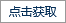匿名?

发表评论

展开全文• ## 最小二乘法系统辨识小结

千次阅读 多人点赞 2019-09-22 15:58:12
系统辨识分为系统结构辨识和系统参数辨识。课程的内容是系统参数的辨识，即假设系统结构已知。 辨识的四要素：输入输出数据，模型集，准则函数，优化方法。 课程中模型的结构已知，实际的辨识中会存在多个可能的...

吴爱国.系统辨识讲义
丁峰.系统辨识新论

最近看完了系统辨识课程的讲义，总结一下讲义上的内容：
系统辨识分为系统结构辨识和系统参数辨识。课程的内容是系统参数的辨识，即假设系统结构已知。
辨识的四要素：输入输出数据，模型集，准则函数，优化方法。
课程中模型的结构已知，实际的辨识中会存在多个可能的模型结构，构成待辨识的模型集。

A ( z ) = 1 + a 1 z − 1 + a 2 z − 2 + . . . . . + a n a z − n a A(z)=1+a_1z^{-1}+a_2z^{-2}+.....+a_{n_a}z^{-n_a} B ( z ) = b 1 z − 1 + b 2 z − 2 + . . . . . + b n b z − n b B(z)=b_1z^{-1}+b_2z^{-2}+.....+b_{n_b}z^{-n_b} C ( z ) = 1 + c 1 z − 1 + c 2 z − 2 + . . . . . + c n c z − n c C(z)=1+c_1z^{-1}+c_2z^{-2}+.....+c_{n_c}z^{-n_c} D ( z ) = 1 + d 1 z − 1 + d 2 z − 2 + . . . . . + d n d z − n d D(z)=1+d_1z^{-1}+d_2z^{-2}+.....+d_{n_d}z^{-n_d} F ( z ) = 1 + f 1 z − 1 + f 2 z − 2 + . . . . . + f n f z − n f F(z)=1+f_1z^{-1}+f_2z^{-2}+.....+f_{n_f}z^{-n_f}
随机白噪声 v ( t ) v(t) , E ( v ( t ) ) = 0 E(v(t))=0 , D ( v ( t ) ) = σ 2 D(v(t))=\sigma^2

# 一.常用随机系统模型

常用的辨识模型有以下几类：

## 1.时间序列模型

（1）自回归模型（AR） A ( z ) y ( t ) = v ( t ) A(z)y(t)=v(t)
该模型表示当前值是过去值的组合。
(2)滑动平均模型(MA) y ( t ) = D ( z ) v ( t ) y(t)=D(z)v(t)
当前值是过去白噪声的组合。
(3)滑动平均自回归模型（ARMA） A ( z ) y ( t ) = D ( z ) v ( t ) A(z)y(t)=D(z)v(t)
（4）确定性模型（ARMA） A ( z ) y ( t ) = B ( z ) u ( t ) A(z)y(t)=B(z)u(t)

## 2.方程误差模型

误差是指描述输入和输出之间的差分方程的误差.
(1)受控自回归模型(ARX) A ( z ) y ( t ) = B ( z ) u ( t ) + v ( t ) A(z)y(t)=B(z)u(t)+v(t)
(2)受控自回归滑动平均模型(ARMAX) A ( z ) y ( t ) = B ( z ) u ( t ) + D ( z ) v ( t ) A(z)y(t)=B(z)u(t)+D(z)v(t)
(3)受控ARAR模型(ARARX) A ( z ) y ( t ) = B ( z ) u ( t ) + v ( t ) / C ( z ) A(z)y(t)=B(z)u(t)+v(t)/C(z)
(4)受控ARARMA模型(ARARMAX) A ( z ) y ( t ) = B ( z ) u ( t ) + D ( z ) C ( z ) v ( t ) A(z)y(t)=B(z)u(t)+\frac{D(z)}{C(z)}v(t)

## 3.输出误差模型

(1)输出误差模型(OE) y ( t ) = B ( z ) A ( z ) u ( t ) + v ( t ) y(t)=\frac{B(z)}{A(z)}u(t)+v(t)
(2)输出误差滑动平均模型(OEMA) y ( t ) = B ( z ) A ( z ) u ( t ) + D ( z ) v ( t ) y(t)=\frac{B(z)}{A(z)}u(t)+D(z)v(t)
(3)输出误差自回归模型(OEAR) y ( t ) = B ( z ) A ( z ) u ( t ) + 1 C ( z ) v ( t ) y(t)=\frac{B(z)}{A(z)}u(t)+\frac{1}{C(z)}v(t)
(4)输出误差自回归滑动平均模型(OEARMA) y ( t ) = B ( z ) A ( z ) u ( t ) + D ( z ) C ( z ) v ( t ) y(t)=\frac{B(z)}{A(z)}u(t)+\frac{D(z)}{C(z)}v(t)

# 二.辨识模型

为了辨识系统参数,一般把系统的参数写成一个参数向量形式,输入输出写成一个信息向量形式,就得到系统的辨识模型,也称为辨识表达式.一般单输入单输出线性参数系统的辨识模型可以表达为: y ( t ) = φ T ( t ) θ + v ( t ) y(t)=\varphi^T(t)\theta+v(t)

# 三. 最小二乘基本原理

以一个典型的受控自回归模型为例:
设系统的模型为 y ( t ) + a 1 y ( t − 1 ) + a − 2 y ( t − 2 ) = b 1 u ( t ) + b 2 u ( t − 1 ) + v ( t ) y(t)+a_1y(t-1)+a-2y(t-2)=b_1u(t)+b_2u(t-1)+v(t)
将其写为如下的形式: y ( t ) = − a 1 y ( t − 1 ) − a 2 y ( t − 2 ) + b 1 u ( t ) + b 2 u ( t − 1 ) + v ( t ) y(t)=-a_1y(t-1)-a_2y(t-2)+b_1u(t)+b_2u(t-1)+v(t)
设观测的数据长度为t,对这个模型有: y ( 3 ) = − a 1 y ( 2 ) − a 2 y ( 1 ) + b 1 u ( 3 ) + b 2 u ( 2 ) + v ( 3 ) y(3)=-a_1y(2)-a_2y(1)+b_1u(3)+b_2u(2)+v(3) y ( 4 ) = − a 1 y ( 3 ) − a 2 y ( 2 ) + b 1 u ( 4 ) + b 2 u ( 3 ) + v ( 4 ) y(4)=-a_1y(3)-a_2y(2)+b_1u(4)+b_2u(3)+v(4) . . . . . . . . . . . . . . . ............... y ( t ) = − a 1 y ( t − 1 ) − a 2 y ( t − 2 ) + b 1 u ( t ) + b 2 u ( t − 1 ) + v ( t ) y(t)=-a_1y(t-1)-a_2y(t-2)+b_1u(t)+b_2u(t-1)+v(t)
Y = [ y ( 3 ) , y ( 4 ) . . . . y ( t ) ] T Y=[y(3),y(4)....y(t)]^T φ T ( i ) = [ − y ( i − 1 ) , − y ( i − 2 ) , u ( i ) , u ( i − 1 ) ] \varphi^T(i)=[-y(i-1),-y(i-2),u(i),u(i-1)] θ = [ a 1 , a 2 , b 1 , b 2 ] \theta=[a_1,a_2,b_1,b_2] H = [ φ ( 3 ) , φ ( 4 ) , . . . . . φ ( t ) ] T H=[\varphi(3),\varphi(4),.....\varphi(t)]^T V = [ v ( 3 ) , v ( 4 ) . . . . v ( t ) ] T V=[v(3),v(4)....v(t)]^T

我们可以得到: Y = H θ + V Y=H\theta+V
令准则函数为 J ( θ ) = ( Y − H θ ) T ( Y − H θ ) J(\theta)=(Y-H\theta)^T(Y-H\theta)
J J θ \theta 求偏导,并令其偏导数为零.得到 θ \theta 的估计值 θ L S = ( H T H ) − 1 H T Y \theta_{LS}=(H^TH)^{-1}H^TY

# 四.最小二乘估计的统计特性

## 1.无偏性定理

已知 θ \theta 的最小二乘估计 θ L S = ( H T H ) − 1 H T Y \theta_{LS}=(H^TH)^{-1}H^TY ,辨识模型可以写成: Y = H θ + W Y=H\theta+W , W W 代表任意形式的噪声.在这里先不考虑 W W 中未知参数的辨识,只考虑与输入和输出(可测)有关的待估计参数.
θ L S \theta_{LS} 的数学期望 E ( θ L S ) = E [ ( H T H ) − 1 H T Y ] E(\theta_{LS})=E[(H^TH)^{-1}H^TY] = E [ ( H T H ) − 1 H T ( H θ + W ) ] θ =E[(H^TH)^{-1}H^T(H\theta+W)]\theta = E [ θ + ( H T H ) − 1 H T W ] =E[\theta+(H^TH)^{-1}H^TW] = θ + E [ ( H T H ) − 1 H T W ] =\theta+E[(H^TH)^{-1}H^TW] 其中 ( H T H ) − 1 H T 为 常 数 (H^TH)^{-1}H^T为常数 ,所以 E ( θ L S ) = θ + ( H T H ) − 1 H T E [ W ] E(\theta_{LS})=\theta+(H^TH)^{-1}H^TE[W] W W 为白噪声,则 E ( θ L S ) = θ E(\theta_{LS})=\theta . E ( θ L S ) E(\theta_{LS}) θ \theta 的无偏估计.
或当噪声向量 W W 的均值为0,且与 H H 无关时, E ( θ L S ) E(\theta_{LS}) θ \theta 的无偏估计.

## 2.估计误差协方差定理

对一个系统 Y = H θ + W Y=H\theta+W ,设噪声向量 W W 的均值为零,协方差矩阵 c o v [ W ] = R v cov[W]=R_v ,且 W W H H 相互独立,则参数估计误差 θ L S ~ = θ L S − θ \tilde{\theta_{LS}}=\theta_{LS}-\theta 的协方差矩阵为 c o v [ θ L S ~ ] = E [ ( H T H ) − 1 H T R v H ( H T H ) − 1 ] cov[\tilde{\theta_{LS}}]=E[(H^TH)^{-1}H^TR_vH(H^TH)^{-1}]
c o v [ θ L S ~ ] = σ 2 E [ ( H T H ) − 1 ] cov[\tilde{\theta_{LS}}]=\sigma^2E[(H^TH)^{-1}]
如果 W W 是一白噪声序列，且其方差为 σ 2 \sigma^2 ,则 c o v [ θ L S ~ ] = σ 2 E [ ( H T H ) − 1 ] cov[\tilde{\theta_{LS}}]=\sigma^2E[(H^TH)^{-1}]

## 3.噪声方差估计定理

对一个系统 Y = H θ + W Y=H\theta+W ,设 W W H H 是统计独立的零均值白噪声向量, W W 的分量为零均值白噪声向量 v ( t ) v(t) ,则v(t)的方差 σ 2 \sigma^2 的估计为: σ 2 ^ = J [ θ L S ~ ] t − n , t 充 分 大 时 \hat{\sigma^2}=\frac{J[\tilde{\theta_{LS}}]}{t-n},t充分大时 其中 n : = d i m θ = θ 的 维 数 n:=dim\theta=\theta的维数

# 五.常用的各类最小二乘辨识算法

## 1.递推最小二乘辨识

适用模型
受控自回归模型(ARX): A ( z ) y ( t ) = B ( z ) u ( t ) + v ( t ) A(z)y(t)=B(z)u(t)+v(t)
将上述模型写为辨识模型的形式: Y = H θ + W Y=H\theta+W
递推最小二乘的算法如下: θ ^ ( t ) = θ ^ ( t − 1 ) + L ( t ) [ y ( t ) − φ T ( t ) θ ^ ( t − 1 ) ] \hat\theta(t)=\hat\theta(t-1)+L(t)[y(t)-\varphi^T(t)\hat\theta(t-1)] L ( t ) = P ( t − 1 ) φ ( t ) 1 + φ T ( t ) P ( t − 1 ) φ ( t ) L(t)=\frac{P(t-1)\varphi(t)}{1+\varphi^T(t)P(t-1)\varphi(t)} P ( t ) = [ I − L ( t ) φ T ( t ) ] P ( t − 1 ) , P ( 0 ) = p 0 I P(t)=[I-L(t)\varphi^T(t)]P(t-1),P(0)=p_0I φ ( t ) = [ − y ( t − 1 ) , − y ( t − 2 ) . . . . , − y ( t − n a ) , u ( t − 1 ) , u ( t − 2 ) , . . . . . u ( t − n b ) ] \varphi(t)=[-y(t-1) ,-y(t-2)....,-y(t-n_a),u(t-1),u(t-2),.....u(t-n_b)]
计算顺序如下:
P ( 0 ) − > L ( 1 ) − > θ ^ ( 1 ) − > P ( 1 ) − > L ( 1 ) − > θ ^ ( 2 ) − > . . . . . P(0)->L(1)->\hat\theta(1)->P(1)->L(1)->\hat\theta(2)->.....
其中P(0)一般取 1 0 6 10^6 数量级.

### 2.带遗忘因子最小二乘系统辨识

适用模型
受控自回归模型(ARX): A ( z ) y ( t ) = B ( z ) u ( t ) + v ( t ) A(z)y(t)=B(z)u(t)+v(t)
将上述模型写为辨识模型的形式: Y = H θ + W Y=H\theta+W .
算法如下:
θ ^ ( t ) = θ ^ ( t − 1 ) + L ( t ) [ y ( t ) − φ T ( t ) θ ^ ( t − 1 ) ] \hat\theta(t)=\hat\theta(t-1)+L(t)[y(t)-\varphi^T(t)\hat\theta(t-1)] L ( t ) = P ( t − 1 ) φ ( t ) λ + φ T ( t ) P ( t − 1 ) φ ( t ) L(t)=\frac{P(t-1)\varphi(t)}{\lambda+\varphi^T(t)P(t-1)\varphi(t)} P ( t ) = 1 λ [ I − L ( t ) φ T ( t ) ] P ( t − 1 ) , P ( 0 ) = p 0 I P(t)=\frac{1}{\lambda}[I-L(t)\varphi^T(t)]P(t-1),P(0)=p_0I
λ \lambda 一般取0.9~1,当 λ \lambda 取1时即为标准最小二乘算法.

### 3.新息与残差之间的关系

新息: e ( t ) = y ( t ) − φ T ( t ) θ ^ ( t − 1 ) e(t)=y(t)-\varphi^T(t)\hat\theta(t-1)
残差: ε ( t ) = y ( t ) − φ T ( t ) θ ^ ( t ) \varepsilon(t)=y(t)-\varphi^T(t)\hat\theta(t)
e ( t ) = [ 1 + 1 λ φ T ( t ) θ ^ ( t ) ] ε ( t ) e(t)=[1+\frac{1}{\lambda}\varphi^T(t)\hat\theta(t)]\varepsilon(t)

### 4.有限数据窗最小二乘系统辨识

适用模型
受控自回归模型(ARX): A ( z ) y ( t ) = B ( z ) u ( t ) + v ( t ) A(z)y(t)=B(z)u(t)+v(t)
将上述模型写为辨识模型的形式: Y = H θ + W Y=H\theta+W .
算法如下:
θ ^ ( t ) = θ ^ ( t − 1 ) + P ( t ) [ φ ( t ) , − φ ( t − p ) ] [ y ( t ) − φ T ( t ) θ ^ ( t − 1 ) , y ( t − p ) − φ T ( t − p ) θ ^ ( t − 1 ) ] T \hat\theta(t)=\hat\theta(t-1)+P(t)[\varphi(t),-\varphi(t-p)][y(t)-\varphi^T(t)\hat\theta(t-1),y(t-p)-\varphi^T(t-p)\hat\theta(t-1)]^T Q ( t ) = P ( t − 1 ) − P ( t − 1 ) φ ( t ) φ T ( t ) P ( t − 1 ) 1 + φ T ( t ) P ( t − 1 ) φ ( t ) Q(t)=P(t-1)-\frac{P(t-1)\varphi(t)\varphi^T(t)P(t-1)}{1+\varphi^T(t)P(t-1)\varphi(t)} P ( t ) = Q ( t ) + Q ( t ) φ ( t − p ) φ T ( t − p ) Q ( t ) 1 − φ T ( t − p ) Q ( t ) φ ( t − p ) P(t)=Q(t)+\frac{Q(t)\varphi(t-p)\varphi^T(t-p)Q(t)}{1-\varphi^T(t-p)Q(t)\varphi(t-p)}

### 5.带遗忘因子的有限数据窗最小二乘算法

适用模型
受控自回归模型(ARX): A ( z ) y ( t ) = B ( z ) u ( t ) + v ( t ) A(z)y(t)=B(z)u(t)+v(t)
将上述模型写为辨识模型的形式: Y = H θ + W Y=H\theta+W .
θ ^ ( t ) = α ( t − 1 ) + P ( t ) φ ( t ) [ y ( t ) − φ T ( t ) α ( t − 1 ) ] \hat\theta(t)=\alpha(t-1)+P(t)\varphi(t)[y(t)-\varphi^T(t)\alpha(t-1)] P ( t ) = 1 λ [ P α ( t − 1 ) − P α ( t − 1 ) φ ( t ) φ T ( t ) P α ( t − 1 ) λ + φ T ( t ) P α ( t − 1 ) φ ( t ) ] P(t)=\frac{1}{\lambda}[P_{\alpha}(t-1)-\frac{P_{\alpha}(t-1)\varphi(t)\varphi^T(t)P_\alpha(t-1)}{\lambda+\varphi^T(t)P_{\alpha}(t-1)\varphi(t)}] α ( t − 1 ) = θ ^ ( t − 1 ) − λ p − 1 P α ( t − 1 ) φ ( t − p ) [ y ( t ) − φ T ( t − p ) θ ^ ( t − 1 ) ] \alpha(t-1)=\hat\theta(t-1)-\lambda^{p-1}P_{\alpha}(t-1)\varphi(t-p)[y(t)-\varphi^T(t-p)\hat\theta(t-1)] P α ( t − 1 ) = P ( t − 1 ) + P α ( t − 1 ) φ ( t − p ) φ T ( t − p ) P α ( t − 1 ) λ − 1 − φ T ( t − p ) P α ( t − 1 ) φ ( t − p ) P_{\alpha}(t-1)=P(t-1)+\frac{P_{\alpha}(t-1)\varphi(t-p)\varphi^T(t-p)P_\alpha(t-1)}{\lambda^{-1}-\varphi^T(t-p)P_{\alpha}(t-1)\varphi(t-p)}展开全文• 最近在做关于过热气温的动态建模问题。有现场运行的历史数据，要找出导...function [sysd,sys,err]=ID(Y,U,Ts)%基于递推最小二乘法参数辨识程序%仅针对二阶系统(工业对象大都是高阶系统，用起来很复杂，所以最终...
•matlab
• 《工业控制计算机》2013 年第 26 卷第 7 期基于最小二乘法的 MATLAB 辨识仿真实验 崔秀美 刘文杰 陈 澄 (苏州大学机电工程学院，江苏 苏州 215021) ExperimentofMATLABIdentificationSimulationBasedonLeastSquares ...
• 控制理论类研究生课程，主要用于模型辨识和组合导航等。
• ## 电池参数辨识之最小二乘法

万次阅读 多人点赞 2018-12-30 16:32:48
锂离子电池研究的流程一般为：实验 ——内部特性研究——建模——参数辨识——估算算法——搭建实验平台。MATLAB
• 二阶RC的带遗忘因子递推最小二乘法ffrls参数辨识，纯代码，代码正确 ID:8350669283870307张徳帅123程序人生
• 基于遗忘因子的最小二乘法电池参数辨识   最小二乘法是系统辨识中最常用的一种估算方法。为了克服最小二乘法存在”数据饱和”的问题，我们通常采用含有遗忘因子的递推最小二乘法(Forgetting Factor Recursive ...
• 最小二乘法最早由高斯在形体运动轨道报告研究工作中提出，此后称为参数辨识理论的基石，被广泛应用于系统辨识和参数估计之中，不得不感叹数学家们的伟大，工程技术的提升离不开这些伟大数学家在工具上的优化。...
• %下面是系统的真实参数 a1 = -1.3;a2 = 0.7; b0 = 1;b1 = -0.5; %下面是采样点的数目: num = 10000;sigma = 1; %下面产生误差 e = normrnd(0,sigma,[num,1]); %下面产生u,y(阶跃响应) u = [zeros(round(num/10),1);...算法 机器学习
• 永磁同步电机的在线参数辨识仿真模型一一一最小二乘法的介绍 1.最小二乘法的简单介绍 最小二乘法的理论最早是由高斯（Gauss）于1795年在星体运动轨道预报研究过程中提出来的。该理论的原理比较简单，容易理解与掌握...
• 最小二乘法（Least Squares Method，简称LS）进行参数估计，首先要指定模型类，通常情况下，采用差分方程来描述被辨识系统，且假定系统的阶次已知。 三、加权最小二乘法 如果考虑到在不同时刻、不同环境下，......